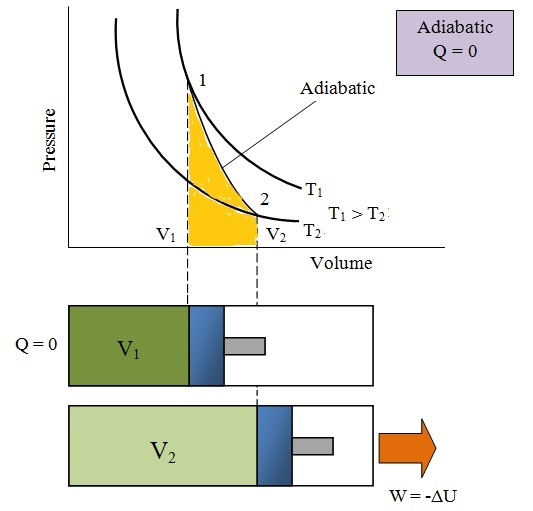A process, in which the working substance neither receives nor gives out heat to its surrounding, during its expansion or compression is called an adiabatic process. This will happens when the working substance remains thermally insulated, so that no heat enters or leaves it during the process. It is thus obvious, that in an adiabatic process:
•  No heat leaves or enters the gas.
• The temperature of the gas changes, as the work is done at the cost of internal energy.
• The change in internal energy is equal to the work done.• P,V,T Relationship:
P1/P2 = (V2/V1) γ
T1/T2 = (V2/V1) γ-1
T1/T2 = (P1/P2) (γ -1)/ γ
• Work done during adiabatic expansion:
W1-2 = (P2V2 - P1V1)/ (γ-1)
= mR (T2-T1)/ (γ-1)
• Change in Internal Energy:
dU = U2-U1 = mCv (T2-T1)
• Heat Supplied or heat transferred:
Q1-2 = 0
• Change in Enthalphy:
dH = H2-H1 = mCp (T2-T1)